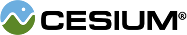#StencilFunction

#### StencilFunction() Scene/StencilFunction.js 15

Determines the function used to compare stencil values for the stencil test.

### Members

#### staticconstantCesium.StencilFunction.ALWAYS : Number Scene/StencilFunction.js 78

The stencil test always passes.

#### staticconstantCesium.StencilFunction.EQUAL : Number Scene/StencilFunction.js 38

The stencil test passes when the masked reference value is equal to the masked stencil value.

#### staticconstantCesium.StencilFunction.GREATER : Number Scene/StencilFunction.js 54

The stencil test passes when the masked reference value is greater than the masked stencil value.

#### staticconstantCesium.StencilFunction.GREATER_OR_EQUAL : Number Scene/StencilFunction.js 70

The stencil test passes when the masked reference value is greater than or equal to the masked stencil value.

#### staticconstantCesium.StencilFunction.LESS : Number Scene/StencilFunction.js 30

The stencil test passes when the masked reference value is less than the masked stencil value.

#### staticconstantCesium.StencilFunction.LESS_OR_EQUAL : Number Scene/StencilFunction.js 46

The stencil test passes when the masked reference value is less than or equal to the masked stencil value.

#### staticconstantCesium.StencilFunction.NEVER : Number Scene/StencilFunction.js 22

The stencil test never passes.

#### staticconstantCesium.StencilFunction.NOT_EQUAL : Number Scene/StencilFunction.js 62

The stencil test passes when the masked reference value is not equal to the masked stencil value.